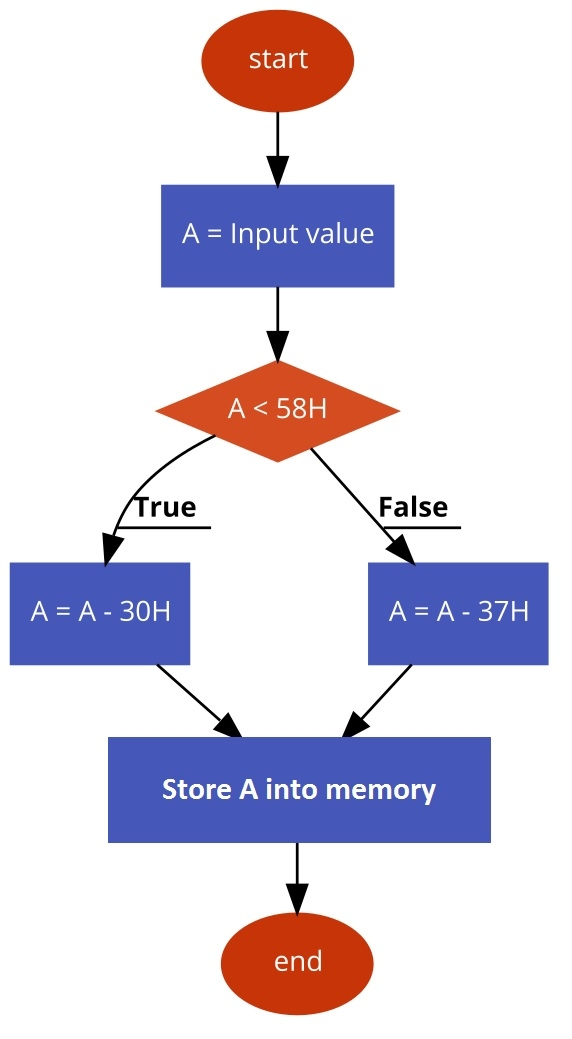# 8085 Program to convert ASCII to HEX

Now let us see a program of Intel 8085 Microprocessor. This program will convert ASCII to HEXvalues.

## Problem Statement

Write 8085 Assembly language program to convert ASCII to Hexadecimal character values.

## Discussion

We know that the ASCII of number 00H is 30H (48D), and ASCII of 09H is39H (57D). So all other numbers are in the range 30H to 39H. The ASCII value of 0AH is 41H (65D) and ASCII of 0FH is 46H (70D), so all other alphabets (B, C, D, E, F) are in the range 41H to 46H.

Here the logic is simple. We are checking whether the ASCII value is less than 58H (ASCII of 9+ 1) When the number is less 58, then it is a numeric value. So we simply subtract 30H from the ASCII value, and when it is greater than58H, then it is alphabetical value. So for that, we are subtracting 37H.

## Input

first input

. . .. . .
800041
. . .. . .

second input

. . .. . .
800035
. . .. . .

third input

. . .. . .
800046
. . .. . .

Flow Diagram## Program

F00021,00, 80

F0037E

MOVA, MLoad ASCII data to Acc from memory
F004FE,58

CPI58HCompare with ASCII(9) + 1
F006D2,0E, F0

JNC NUMThe input is numeric
F009D6,37

SUI 37HSubtract offset to get Alphabetic character
F00BC3,10, F0

JMP STOREStore the result
F00ED6,30NUMSUI 30HSubtract 30 to get the numeric value
F01023STOREINX HPoint to next location
F01177

MOVM, AStore Acc content to memory
F01276

HLTTerminate the program

first output

. . .. . .
80010A
. . .. . .

second output